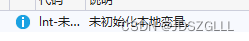# C++超詳細梳理基礎知識

## 命名空間的使用

### 來源

C++是在C的基礎上發展而形成的一種語言，完全兼容C的語法，也加入瞭許多新的規則和語法來解決C的缺陷。

```#include<stdio.h>
int main()
{
int scanf = 20;
printf("%d", scanf);
return 0;
}``````#include<stdio.h>
int main()
{
int scanf = 20;
scanf("%d", &scanf);
printf("%d", scanf);
return 0;
}```

### 命名空間的使用

```#include<iostream>
using namespace std;
int main()
{
cout << "hello  world" << endl;
return 0;
}```

```#include<iostream>
using namespace std;
namespace  N1
{
int printf = 30;
int strlen = 20;
}
int main()
{
cout << "hello  world" << endl;
cout << N1::printf << endl;
return 0;
}```

```namespace  N1
{
int printf = 30;
int strlen = 20;
namespace  N2
{
int a = 0;
}
}```

```int main()
{
std::cout << "hello  world" << std::endl;
//cout << N1::printf << endl;
return 0;
}```

`using namespace std;`

using的作用是把命名空間中的內容在全局空間中展開，命名空間中的變量就成為瞭全局變量，調用時就不需要命名空間名加上作用域限定符瞭。

#### 不展開

`std::cout << "hello  world" << std::endl;`

#### 部分展開

```using std::cout;
using std::endl;
int main()
{
cout << "hello  world" << endl;
//cout << N1::printf << endl;
return 0;
}```

#### 全展開

```using namespace std;
int main()
{
cout << "hello  world" << endl;
//cout << N1::printf << endl;
return 0;
}```

### 函數重載

#### 函數重載的規則

```int Add(int left, int right)
{
return left+right;
}
{
return left+right;
}
{
return left+right;
}
int main()
{
return 0;
}```

```short Add(short left, short right)
{
return left+right;
}
{
return left+right;
}```

#### C++如何實現函數重載C和C++的區別在於，在編譯的符號匯總中，C語言是使用函數的原名進行匯總的，導致瞭一個名稱隻能對應一個函數，所以不能進行函數重載。

```int Add(int left, int right)
{
return left+right;
}

{
return left+right;
}

{
return left+right;
}```

## 引用

```#include<iostream>
int main()
{
int a = 0;
int b = 0;
int& c = a;
c = 10;
return 0;
}```1.引用類型必須和引用實體是同種類型的2.引用在定義時必須初始化

```int a;
int& b = a;```3.一個變量可以有多個引用

```int a = 0;
int& b = a;
int& c = a;
int\$ d = c;```

4.引用一旦引用一個實體，再不能引用其他實體

```#include<iostream>
int main()
{
int a = 0;
int b = 0;
int& c = a;
int& c = b;
c = 10;
return 0;
}```